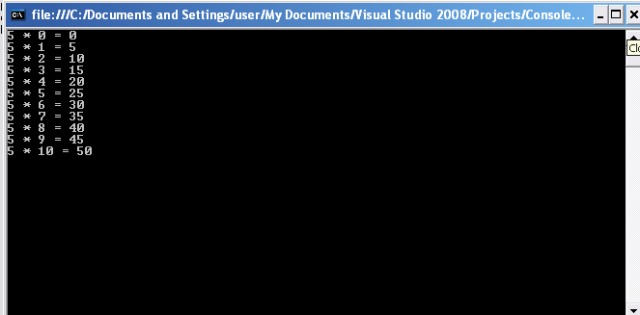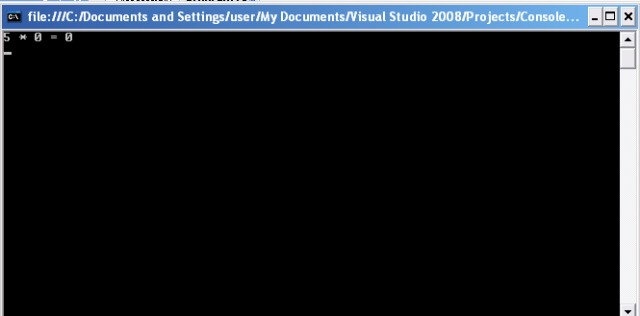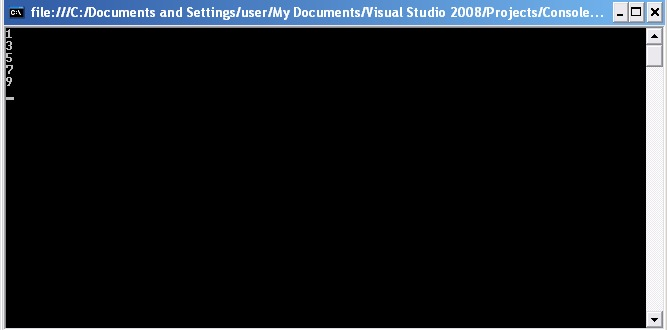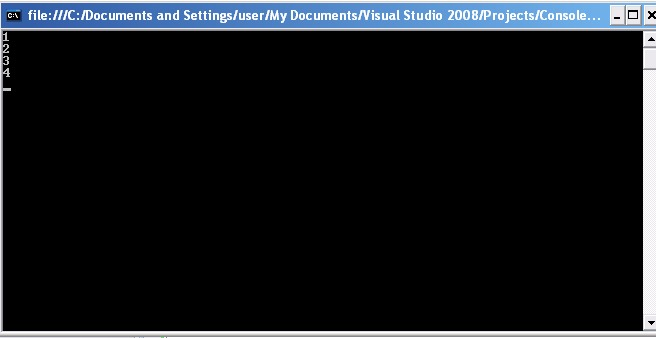In previous tutorial we learned about conditional and unconditional statements, now we will look about iterative statements like while, for, goto, break etc.

## 1. goto statement:

It is the basic and simple of all the iterative statements. For using it, we need to create a label, and then further in the program call that label. A label is identified by a name followed by the colon(:), And for calling this label goto keyword is used which is followed by the label name.

Example: The following code snippet displays table of 5 using goto statement.

int a = 5, b= 0;
int result;
come_here:                            //label
result = a * b;
Console.WriteLine(a+” * “+b + ” = “+result);
b++;
if(b<=10)
goto come_here;                      //calling label

Output:## 2. while loop:

The while in normal language can be said as “while this condition is true execute the statement block“.

Syntax:

while(expression)

{statements}

In syntax, the expression is the condition that is checked and based on the value that is true or false. The block of statements are executed.

Example:The example is same as that of goto statement i.e of table of 5, using while loop.

int a = 5, b= 0;                              //initializing variables
int result;
while (b <= 10)                            //condition checking
{
result = a * b;
Console.WriteLine(a + ” * ” + b + ” = ” + result);
b++;                                   //Incrementing counter/variable
}

Output: Same as previous…

## 3. do…while loop:

It is another variant of while loop, In while loop, the statements will never execute if condition is false, However if we want the statements to get executed at-least once, then we need to use do…while loop.

Syntax:

do

{statements}

while(expression)

As seen from the syntax firstly the statements are executed and then the condition(expression) is checked, if it is true then same block is again executed or else it is skipped. That means in any case it is at-least executed once.

Example:

1.

int a = 5, b= 0;
int result;
do
{
result = a * b;
Console.WriteLine(a + ” * ” + b + ” = ” + result);
b++;
} while (b > 10);In the above code snippet the do..while block is executed once and then condition is checked and the expression evaluated false, thus by skipping the statement.

2.

int a = 5, b= 0;
int result;
do
{
result = a * b;
Console.WriteLine(a + ” * ” + b + ” = ” + result);
b++;
} while (b <= 10);

The above code snippet displays the table of 5, as like in output
of goto example.

## 3. for loop:

If you have carefully noted the while loop code snippet, first the variables are initialized, condition is specified and then the counter or  variable is incremented. All this is done in three different statements. The same condition can be written using for loop in a single statement.

Syntax:

for ([initializers]; [expression]; [iterators])

{statement}

For loop statement consists of three parts separated by semicolon , initializers used for variable initializing, second expression used for specifying condition and thirdly iterators used for incrementing counter, All these three parts are optional.

Example:

1.

for (int a= 5,b=0,result ; b<=10 ; b++ )
{
result = a * b;
Console.WriteLine(a + ” * ” + b + ” = ” + result);
}

2.

int a = 5, b = 0, result;
for ( ; b<=10 ; b++ )
{
result = a * b;
Console.WriteLine(a + ” * ” + b + ” = ” + result);
}

3.

for (int a= 5,b=0,result ; b<=10 ; )
{
result = a * b;
Console.WriteLine(a + ” * ” + b + ” = ” + result);
b++;
}

4.

for (int a= 5,b=0,result ;  ; b++ )
{
if (b <= 10)
{
result = a * b;
Console.WriteLine(a + ” * ” + b + ” = ” + result);
}
else
break;
}

5.

for (int a= 5,b=0,result ;  ; )
{
if (b <= 10)
{
result = a * b;
Console.WriteLine(a + ” * ” + b + ” = ” + result);
}
else
break;
b++;
}

6.

int a = 5, b = 0, result;
for ( ; ; )
{
if (b <= 10)
{
result = a * b;
Console.WriteLine(a + ” * ” + b + ” = ” + result);
}
else
break;
b++;
}

All the above six code snippets display the same output(table of 5) using combinations in for loop.

## 4.foreach loop:

This loop is similar to for loop, but it works only with array and is auto incremented by 1.

Example:

int[] result  = new int;
int a = 5;
int b = 0;
foreach (int i in result)
{
result[i]= a * b;
Console.WriteLine(a + ” * ” + b + ” = ” + result[i]);
b++;
}

In the above code snippet a array of result is created and the table of 5 is stored in that array as well as it is also simultaneously displayed using foreach loop.

## 5. continue and break:

Many a times we need to return to the top of the loop, without further executing the statements in the loop. The continue statement returns to the top of the loop and skips the execution of the remaining statements.

Example:

for (int i = 1; i <= 10; i++)
{
if (i%2==0)
{
continue;
}
Console.WriteLine(i);
}

Output:In this example, a counter is initialized to count from 1 to 10. By using the continue statement in conjunction with the expression `(i%==0)it checks for even numbers`, the statements between continue and the end of the for body are skipped for every even number, thus only odd numbers are displayed.

The opposite to continue is the break, as the name implies it breaks out of the loop and terminates all execution of the loop irrespective of the condition.

Example:

for (int i = 1; i <= 10; i++)
{
if (i==5)
{
break;
}
Console.WriteLine(i);
}

Output:By now you must have known about all the types of statements and their execution flow, in the next tutorials we will start with the Object oriented features of C sharp.

Share.